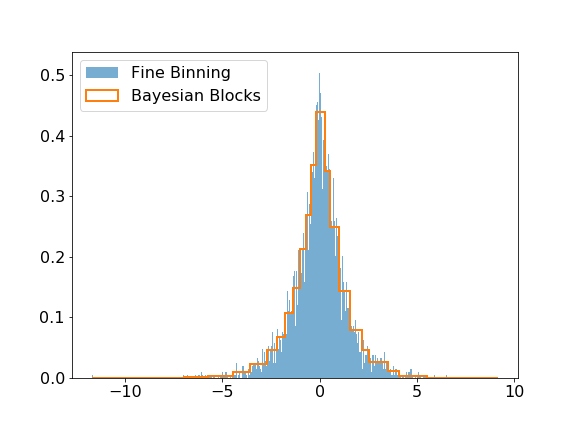# modeling#

The modeling submodule includes the Bayesian Block algorithm that can be used to improve the binning of histograms. The visual improvement can be dramatic, and more importantly, this algorithm produces histograms that accurately represent the underlying distribution while being robust to statistical fluctuations. Here is a small example of the algorithm applied on Laplacian sampled data, compared to a histogram of this sample with a fine binning.

```>>> import numpy as np
>>> import matplotlib.pyplot as plt
>>> from hepstats.modeling import bayesian_blocks

>>> # sample data from a Laplacian distribution
>>> data = np.random.laplace(size=10000)
>>> blocks = bayesian_blocks(data)

>>> # plot the histograms of the data with 1000 equally spaced bins and the bins from the
>>> # bayesian_blocks function
>>> plt.hist(data, bins=1000, label='Fine Binning', density=True, alpha=0.6)
>>> plt.hist(data, bins=blocks, label='Bayesian Blocks', histtype='step', density=True,
>>>          linewidth=2)
>>> plt.legend(loc=2)
```